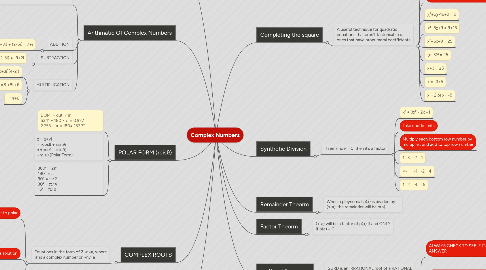# Complex NumbersAlesha Neame
Get Started. It's FreeComplex Numbers## 2. Arithmatic Of Complex Numbers

### 2.1. CONJUGATE COMPLEX NUMBERS

2.1.1. MULTIPLY BY THE CONJUGATE TO DIVIDE

2.1.2. If z = a-bi, then z̅ =a + bi

2.1.3. (14+8i) ÷ (2+3i)

2.1.4. ((14+8i) ÷ (2+3i)) ×((2-3i) ÷(2-3i)) (FOILS AGAIN, WRITE AS FRACTION

2.1.5. = 4-2i

2.2.1. (2+3i) + (2-5i) = 7+i

### 2.3. SUBTRACTION

2.3.1. (-7-3i) - (2-5i) = -9+2i

### 2.4. MULTIPLICATION

2.4.1. (2+3i)(4-2i)

2.4.2. =8 +8i +6

2.4.3. =14+6

## 3. Completing the square

### 3.1. A useful technique for quadratic equations that aren't factorisable or ones that have pronumeral coefficients

3.1.1. χ² + 6χ -16 = 0

3.1.2. Take co-efficient of χ, half it, square it and add result to both sides

3.1.2.1. 6 ÷ 2 = 3

3.1.2.2. 3² = 9

3.1.3. χ²+6χ-16+9 = 9

3.1.4. x²+6χ+9 = 9+16

3.1.5. x²+6x+9 = 25

3.1.6. (x+3)² = 25

3.1.7. x+3 = ±5

3.1.8. x = -3±5

3.1.9. x = 2 or x= -8

## 4. Synthetic Division

### 4.1. If remainder =0, then it’s a factor

4.1.1. x³ + 3x² - 2x +1

4.1.2. Take coefficients

4.1.3. Multiply each bottom row number by multiplier, and add to top row number

4.1.4. 1 3 -2 1

4.1.5. ×-1 -1 -2 4

4.1.6. 1 2 -4 r5

## 7. Irrational Equations

### 7.1. SURD is an IRRATIONAL root of a RATIONAL number

7.1.1. ALWAYS CHECK TO SEE IF ITS AN ANSWER

7.1.2. CONJUGATE SURDS is if a+√b is a complex SURD, then it's conjugate is a−√b

7.1.2.1. MULTIPLYING & DIVIDING √a√b = √a×b √a÷√b = √a÷b e.g (2+2√3)(√15-√12) =2√15 - 2√12 + 2√3 + √15 − 2√3√12 (FOILS) =2√15 − 2√12 + 2√45 - 2√36 =2√15 - 2√4√3 + 2√9√5 − 12 =2√15 - 4√3 - 6√5 − 12

## 9. COMPLEX ROOTS

### 9.1. Equations in the form of Z¬n=a, where ’a’ is a complex number or ¬n√a

9.1.1. 1- Convert to polar

9.1.1.1. Z³ = -8

9.1.1.2. Z³ = 8cis180°

9.1.2. 2 - Let rcisθ be a solution

9.1.2.1. (rcisθ)³ = 8cis180°

9.1.2.2. r³cis3θ = 8cis180°

9.1.2.3. r³ = 8 and 3θ = 180

9.1.2.4. 2 = 2 and θ = 60

9.1.3. Find number of soln. according to exponent thing (Z³ so 3 soln). All solutions are evenly spaced around argan diagram